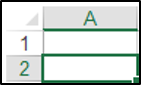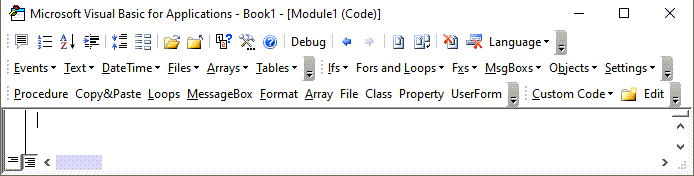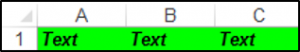# VBA Select Range / Cells

VBA allows you to select a cell, ranges of cells, or all the cells in the worksheet. You can manipulate the selected cell or range using the Selection Object.

## Select a Single Cell Using VBA

You can select a cell in a worksheet using the Select method. The following code will select cell A2 in the ActiveWorksheet:

``Range("A2").Select``

Or

``Cells(2, 1).Select``

The result is:## Select a Range of Cells Using VBA

You can select a group of cells in a worksheet using the Select method and the Range object. The following code will select A1:C5:

``Range("A1:C5").Select``

## Select a Range of Non-Contiguous Cells Using VBA

You can select cells or ranges that are not next to each other, by separating the cells or ranges using a comma in VBA. The following code will allow you to select cells A1, C1, and E1:

``Range("A1, C1, E1").Select``

You can also select sets of non-contiguous ranges in VBA. The following code will select A1:A9 and B11:B18:

``Range("A1:A9, B11:B18").Select``

## Select All the Cells in a Worksheet

You can select all the cells in a worksheet using VBA. The following code will select all the cells in a worksheet.

``Cells.Select``

## Select a Row

You can select a certain row in a worksheet using the Row object and the index number of the row you want to select. The following code will select the first row in your worksheet:

``Rows(1).Select``

## Select a Column

You can select a certain column in a worksheet using the Column object and the index number of the column you want to select. The following code will select column C in your worksheet:

``Columns(3).Select``

## VBA Coding Made Easy

Stop searching for VBA code online. Learn more about AutoMacro - A VBA Code Builder that allows beginners to code procedures from scratch with minimal coding knowledge and with many time-saving features for all users!## Select the Last Non-Blank Cell in a Column

Let’s say you have data in cells A1, A2, A3 and A4 and you would like to select the last non-blank cell which would be cell A4 in the column. You can use VBA to do this and the Range.End method.

The Range.End Method can take four arguments namely: xlToLeft, xlToRight, xlUp and xlDown.

The following code will select the last non-blank cell which would be A4 in this case, if A1 is the active cell:

``Range("A1").End(xlDown).Select``

## Select the Last Non-Blank Cell in a Row

Let’s say you have data in cells A1, B1, C1, D1, and E1 and you would like to select the last non-blank cell which would be cell E1 in the row. You can use VBA to do this and the Range.End method.

The following code will select the last non-blank cell which would be E1 in this case, if A1 is the active cell:

``Range("A1").End(xlToRight).Select``

## Select the Current Region in VBA

You can use the CurrentRegion Property of the Range Object in order to select a rectangular range of blank and non-blank cells around a specific given input cell. If you have data in cell A1, B1 and C1, the following code would select this region around cell A1:

``Range("A1").CurrentRegion.Select``

So the range A1:C1 would be selected.

VBA Programming | Code Generator does work for you!

## Select a Cell That is Relative To Another Cell

You can use the Offset Property to select a cell that is relative to another cell. The following code shows you how to select cell B2 which is 1 row and 1 column relative to cell A1:

``Range("A1").Offset(1, 1).Select``

## Select a Named Range in Excel

You can select Named Ranges as well. Let’s say you have named cells A1:A4 Fruit. You can use the following code to select this named range:

``Range("Fruit").Select``

## Selecting a Cell on Another Worksheet

In order to select a cell on another worksheet, you first need to activate the sheet using the Worksheets.Activate method. The following code will allow you to select cell A7, on the sheet named Sheet5:

``````Worksheets("Sheet5").Activate
Range("A1").Select``````

## Manipulating the Selection Object in VBA

Once you have selected a cell or range of cells, you can refer to the Selection Object in order to manipulate these cells. The following code selects the cells A1:C1 and sets the font of these cells to Arial, the font weight to bold, the font style to italics and the fill color to green.

``````Sub FormatSelection()
Range("A1:C1").Select

Selection.Font.Name = "Arial"
Selection.Font.Bold = True
Selection.Font.Italic = True
Selection.Interior.Color = vbGreen

End Sub``````

The result is:## Using the With…End With Construct

We can repeat the above example using the With / End With Statement to refer to the Selection Object only once. This saves typing and usually makes your code easier to read.

``````Sub UsingWithEndWithSelection()
Range("A1:C1").Select

With Selection
.Font.Name = "Arial"
.Font.Bold = True
.Font.Italic = True
.Interior.Color = vbGreen
End With

End Sub``````